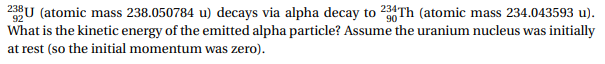# 23U (atoic mass 238.050784 u) decays via alpha decay to 234Th (atomic mass 234.043593 u). What is the kinetic energy of the emitted alpha particle? Assume the uranium nucleus was initially at rest (so the initial momentum was zero).

Questionhelp_outlineImage Transcriptionclose23U (atoic mass 238.050784 u) decays via alpha decay to 234Th (atomic mass 234.043593 u). What is the kinetic energy of the emitted alpha particle? Assume the uranium nucleus was initially at rest (so the initial momentum was zero). fullscreen

### Want to see the step-by-step answer?